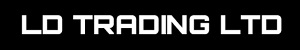# Dealer Details# Welcome to L D Trading Ltd

Below you will find a range of premium history checked vehicles we have available.

… of 2
£1,995
J U S T B E E N S E R V I C E D + W A R R A N T Y
•1.6L
• 57.5kMiles
•Diesel
•Manual
•Coupe

£3,795
J U S T B E E N S E R V I C E D + W A R R A N T Y
•1.4L
• 79.2kMiles
•Diesel
•Manual
•Hatchback

£3,950
J U S T B E E N S E R V I C E D + W A R R A N T Y
•1.6L
• 65.1kMiles
•Petrol
•Manual
•Estate

£3,995
F S H + N E W B E L T + N E W T Y R E S + W A R R A N T
•1.8L
• 77.6kMiles
•Petrol
•Manual
•Estate

£4,295
J U S T B E E N S E R V I C E D + W A R R A N T Y
•1.8L
• 52.1kMiles
•Petrol
•Manual
•People Carrier

£4,500
O n e O w n e r F r o m N e w + W a r r a n t y
•2L
• 88.8kMiles
•Diesel
•Manual
•SUV

£4,500
O n e O w n e r F r o m N e w + W a r r a n t y
•2L
• 48.3kMiles
•Petrol
•Manual
•Estate

£4,695
F U L L F O R D S E R V I C E H I S T O R Y
•2.2L
• 94.7kMiles
•Diesel
•Manual
•People Carrier

£4,795
F U L L S E R V I C E H I S T O R Y + W A R R A N T Y
•2L
• 83.5kMiles
•Diesel
•Manual
•Convertible

£4,795
J U S T B E E N S E V I C E D + W A R R A N T Y
•2L
• 92.6kMiles
•Diesel
•Manual
•Saloon

£4,795
O n e O w n e r , F S H , F r e e W a r r a n t y
•1.4L
• 60kMiles
•Petrol
•Manual
•Hatchback

£4,995
J u s t B e e n S e r v i c e d + W a r r a n t y +
•2L
• 58.5kMiles
•Diesel
•Semi Auto
•Hatchback

£5,000
O n e O w n e r F r o m N e w + W a r r a n t y
•2.2L
• 84.4kMiles
•Diesel
•Manual
•People Carrier

£5,195
J U S T B E E N S E R V I C E D + W A R R A N T Y
•1.6L
• 68.9kMiles
•Petrol
•Manual
•Hatchback

£5,395
J u s t B e e n S e r v i c e d + W a r r a n t y
•2.1L
• 89kMiles
•Diesel
•Manual
•Saloon